# Predicate calculus

(diff) ← Older revision | Latest revision (diff) | Newer revision → (diff)

A formal axiomatic theory; a calculus intended for the description of logical laws (cf. Logical law) that are true for any non-empty domain of objects with arbitrary predicates (i.e. properties and relations) given on these objects.

In order to formulate the predicate calculus one must first fix an exact logico-mathematical language. In the most common case of single-sorted first-order languages, such a language contains predicate variables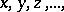function symbols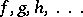with a varying number of argument places, and predicate symbols (predicate letters)also with a varying number of argument places. From the variables and function symbols one constructs the terms of the language, which are names of objects of the theory to be studied. Further, ifis an-place predicate symbol of,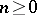, and if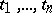are terms, thenis, by definition, an atomic (elementary) formula of. The content of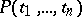is that the statement according to whichare related through the relationis true.

By means of propositional connectives (cf. Propositional connective) and quantifiers (cf. Quantifier) one constructs formulas of the language from the atomic formulas. The common choice of connectives and quantifiers in classical and intuitionistic predicate calculus is:or(conjunction, "and" ),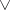(disjunction, non-exclusive "or" ),or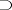(implication, "implies" , "if … then" ),(negation, "not" ),(the universal quantifier, "for all" ), and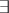(the existential quantifier, "there exists" ). The corresponding non-atomic formulas of these calculi have the form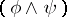,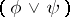,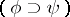,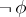,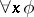,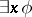. An occurrence of a variablein a formulais called bound ifoccurs in a part ofof the formor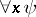. All other occurrences ofinare called free. A variableis called a parameter ofif there is at least one free occurrence ofin. Intuitively speaking, a formula with parameters expresses a condition that is turned into a concrete statement if a model of the calculus is given, i.e. some non-empty domain of objects of study is chosen and predicates (i.e. relations on the domains of the objects) are ascribed to the predicate symbols, while the parameters are ascribed definite objects as values.

A predicate calculus is specified by means of axioms and derivation rules. E.g., one of the usual formulations of classical predicate calculus contains the axioms

1)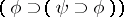;

2)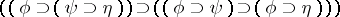;

3)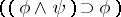;

4)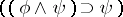;

5)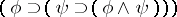;

6);

7);

8)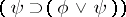;

9)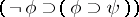;

10)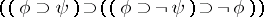;

11);

12)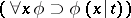;

13)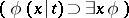;

14)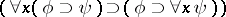;

15)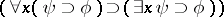. Here,,anddenote arbitrary formulas of, so that each of 1)–15) represents an axiom scheme generating a concrete axiom of the calculus for a concrete choice of,and. Further, in 14) and 15) it is assumed thatis not a parameter of; in 12) and 13)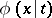denotes the result of simultaneous substitution of all free occurrences ofinby the term. (Moreover, ifshows up instead ofin a part ofof the form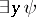or, whereoccurs in, then one must additionally replace all bound occurrences ofin this part by a variable not occurring in; this is done in order not to distort the meaning ofwhen replacingwith; such a distortion of meaning is called collision of variables.)

Further, predicate calculus contains two derivation rules: a) if formulasandhave been derived, then a derivation forexists (the rule of modus ponens); and b) if a formulahas been derived and ifis a variable, then a derivation forexists (the rule of generalization).

The interpretation of the logical connectives in predicate calculus is the same as in propositional calculus. In connection with the interpretation of quantifiers, it should be noted that in classical predicate calculus they are treated with the use of the actual infinity. If an interpretation of the language is given, then every formula not containing parameters receives the value "true" or "false" . A formula is called classically (universally) valid if in any interpretation and for all values ascribed to the parameters, it has the value "true" . By virtue of the Gödel completeness theorem, the classically-valid formulas are exactly those which can be derived in classical predicate calculus. This theorem is an exact expression of the idea of a formalization of classical logic: In classical predicate calculus all logical laws that are true in all models can be derived.

Intuitionistic predicate calculus differs from classical predicate calculus in that axiom scheme 11) does not occur in its list of axiom schemes. The difference between the two calculi is reflected in the way the logical connectives and quantifiers are understood. In intuitionistic predicate calculus this understanding is within the framework of intuitionism. The question of the completeness of intuitionistic predicate calculus is much more complicated and admits different solutions, depending on the particular semantics; but here too one can develop a very substantial theory of models, analogous to classical model theory (cf. Kripke models; Realizability).

One also uses other formulations of predicate calculus, the most important of which, from the point of view of proof theory, are the calculus of natural deduction (cf. Natural logical deduction) and the sequent calculi (cf. Sequent calculus).

Predicate calculus is a very common basis for the construction of logical calculi intended for the description of fragments of some concrete mathematical theory. E.g., if one wishes to describe some class of true statements of set theory, then one can construct logical calculi in terms of set theory in which, apart from the axioms and derivation rules of classical predicate calculus (the logical postulates), additional non-logical axioms will figure, describing properties of sets. The axiom of choice is a typical example of a non-logical axiom in set theory.

Predicate calculus is sometimes called narrow predicate calculus, first-order predicate calculus or first-order functional calculus, as distinct from calculi containing quantifiers over predicates and corresponding convolution axioms, expressing the existence of the respective predicates. Such a kind of calculus, which already does not have a pure logical character, is often called a higher-order predicate calculus. The theory of types is an example of such a calculus (cf. Types, theory of). Besides classical and intuitionistic predicate calculus there are other types of logical systems describing logical laws which can be expressed by other logical tools or from other methodological positions. Amongst these are modal predicate calculus, inductive logic, etc.

How to Cite This Entry:
Predicate calculus. Encyclopedia of Mathematics. URL: http://encyclopediaofmath.org/index.php?title=Predicate_calculus&oldid=16765
This article was adapted from an original article by A.G. Dragalin (originator), which appeared in Encyclopedia of Mathematics - ISBN 1402006098. See original article